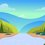# Proof that the shortest curve joining two points is a straight line.

Let the two points be labelled $A$ and $B$ and have coordinates $(a,y(a))$ and $(b,y(b))$. The length of an element of path $ds$ is given by $ds=\sqrt{dx^2+dy^2} = \sqrt{1+y'^2}\;dx$ and hence the total path length is given by $L=\int_a^b{ \sqrt{1+y'^2}} \;dx.$ The Euler-Lagrange equation $\frac{\partial F}{\partial y} = \frac{d}{dx}\left(\frac{\partial F}{\partial y'} \right)$ can be rearranged to $\frac{\partial F}{\partial y'} = \mathrm{const.}$ since $F= \sqrt{1+y'^2}$ does not contain $y$ explicity. We can now differentiate $F$ and set it to a constant value. $k=\frac{\partial F}{\partial y'} = \frac{y'}{\sqrt{1+y'^2}}$ $k^2(1+y'^2) = y'^2$ $k^2 = y'^2-k^2y'^2 = y'^2(1-k^2)$ $\Rightarrow y'=\frac{k}{\sqrt{1-k^2}}$ Integration gives $y(x) = \frac{k}{\sqrt{1-k^2}}x+c$/extract_itex] which, as expected, is the equation of a straight line in the form $y=mx+c$. $\;\;\;\;\;\;\;\;\;\;\;\;\;\;\;\;\;\;\;\;\;\;\;\;\;\;\;\;\;\;\;\;\;\;\;\;\;\;\;\;\;\;\;\;\;\;\;\;\;\;\;\;\;\;\;\;\;\;\;\;\;\;\;\;\;\;\;\;\;\;\;\;\;\;\;\;\;\;\;\;\;\;\;\;\;\;\;\;\;\;\;\;\;\;\;\;\;\;\;\;\;\;\;\;\;\;\;\;\;\;\;\;\;\;\;\;\;\;\;\;\;\;\;\;\;\;\;\;\;\;\;\;\;\;\;\;\;\;\;\;\;\;\;\;\;\;\;\;\;\;\;\;\;\;\;\;\;\;\;\;\Box.$Note by Samuel Braun 3 months, 2 weeks ago This discussion board is a place to discuss our Daily Challenges and the math and science related to those challenges. Explanations are more than just a solution — they should explain the steps and thinking strategies that you used to obtain the solution. Comments should further the discussion of math and science. When posting on Brilliant: • Use the emojis to react to an explanation, whether you're congratulating a job well done , or just really confused . • Ask specific questions about the challenge or the steps in somebody's explanation. Well-posed questions can add a lot to the discussion, but posting "I don't understand!" doesn't help anyone. • Try to contribute something new to the discussion, whether it is an extension, generalization or other idea related to the challenge. • Stay on topic — we're all here to learn more about math and science, not to hear about your favorite get-rich-quick scheme or current world events. MarkdownAppears as *italics* or _italics_ italics **bold** or __bold__ bold - bulleted- list • bulleted • list 1. numbered2. list 1. numbered 2. list Note: you must add a full line of space before and after lists for them to show up correctly paragraph 1paragraph 2 paragraph 1 paragraph 2 [example link](https://brilliant.org)example link > This is a quote This is a quote  # I indented these lines # 4 spaces, and now they show # up as a code block. print "hello world" # I indented these lines # 4 spaces, and now they show # up as a code block. print "hello world" MathAppears as Remember to wrap math in $$ ... $$ or \[ ... $ to ensure proper formatting.
2 \times 3 $2 \times 3$
2^{34} $2^{34}$
a_{i-1} $a_{i-1}$
\frac{2}{3} $\frac{2}{3}$
\sqrt{2} $\sqrt{2}$
\sum_{i=1}^3 $\sum_{i=1}^3$
\sin \theta $\sin \theta$
\boxed{123} $\boxed{123}$

Sort by:

You should mention that the curve is confined to a plane. Such curves are called geodesics, and have different shapes on different surfaces. On the surface of a sphere for example, it is the great circle of the sphere. As you are including $x$ and $y$ only, it refers to a plane curve.

- 3 months, 1 week ago